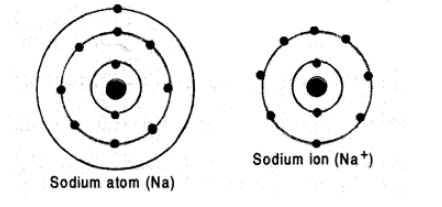# Show diagramatically the electronic`
Question:

Show diagramatically the electronic distribution in a sodium atom and a sodium ion and also give their atomic number.

Solution:

The atomic number of sodium (Na) is 11 . The electronic distribution in the atom is $K(2) L(8)$ and $L(1)$.

Sodium

ion (Na^t) is formed by removal of one electron from the atom. Its electronic distribution is $K(2)$ and $L(8)$.

This means that $N a^{+}$ion has the elecronic configuration of Ne atom which is inert gas atom. The electronic

distribution of the atom and ion are shown as follows: